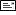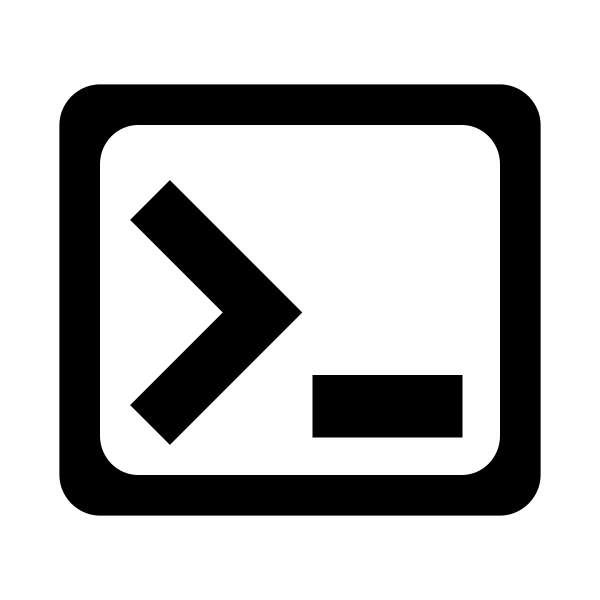# Python2VB Beta 0.11

Python to Visual Basic source code converter

### Description

Python2VB converts Python source code into Visual Basic code.
This is a development version so beware of many error messages.

### Syntax

 Keywords Python Vb.Net Remarks """Example Do addition. """ ' Example ' Do addition. ' Subroutines def main():     winWidth = 300 main() Public Sub main()     winWidth = 300 End Sub main() Variables winWidth = 300 Dim winWidth As Integer winWidth = 300 GraphWin win = GraphWin("Addition", winWidth, winHeight) Form1.Text = "Addition" Form1.Width = winWidth + 100 Form1.Height = winHeight + 100 setCoords win.setCoords(0,0, winWidth, winHeight) (Used by Python2VB) p2vbX1 = 0 p2vbY1 = 0 p2vbX2 = winWidth p2vbY2 = winHeight Text i = Text(Point(w, 30), "Enter two numbers.") p2vbW = winWidth p2vbH = winHeight Dim instructions As New Label i.Left = p2vbW * ((w) / (p2vbX2 - p2vbX1)) i.Top = p2vbH - (p2vbH * (( 30) / (p2vbY2 - p2vbY1))) i.Text = "Enter two numbers." i.AutoSize = True

If you want a file converted, you canit to me.

You can support Python2VB with this Paypal link.